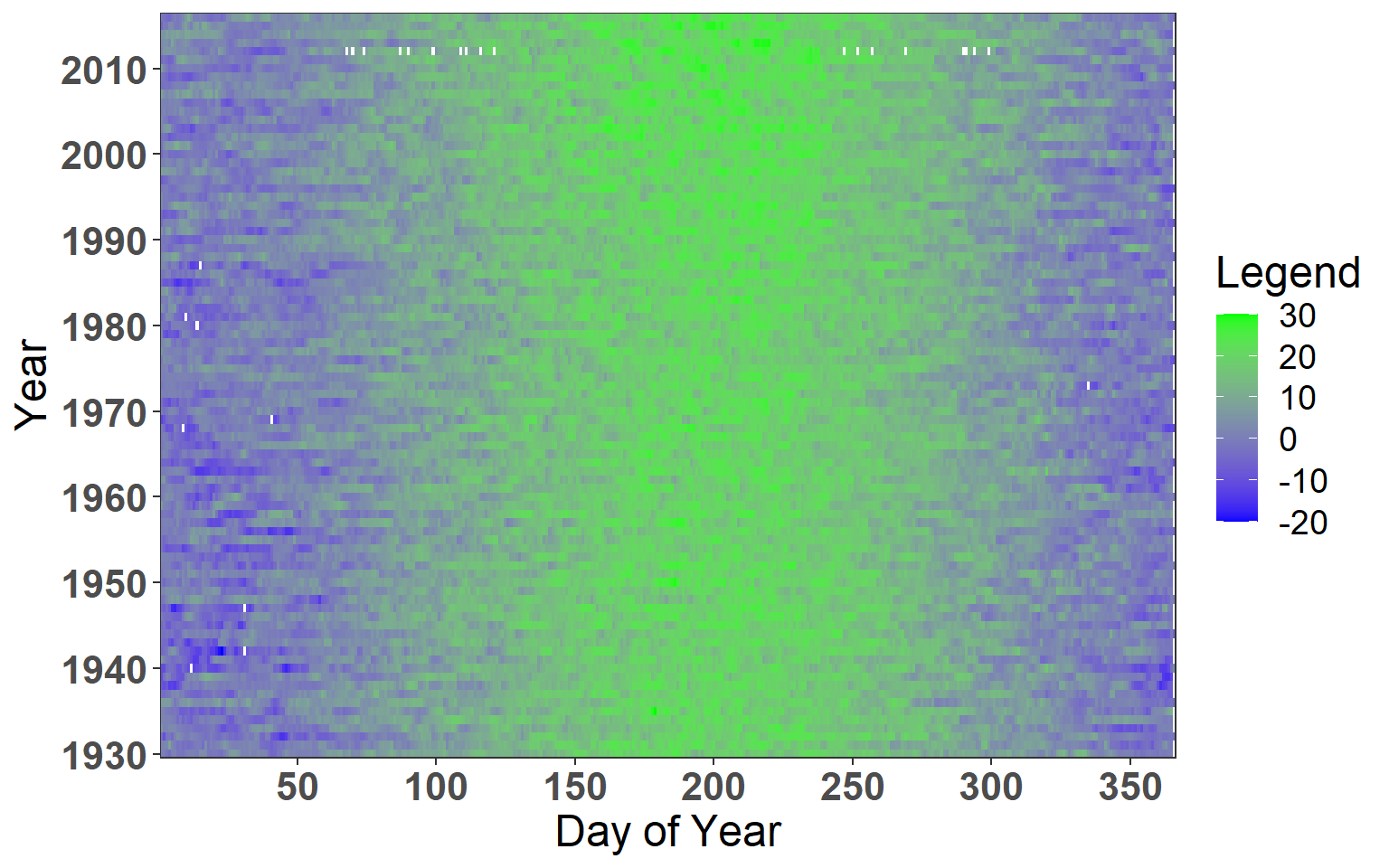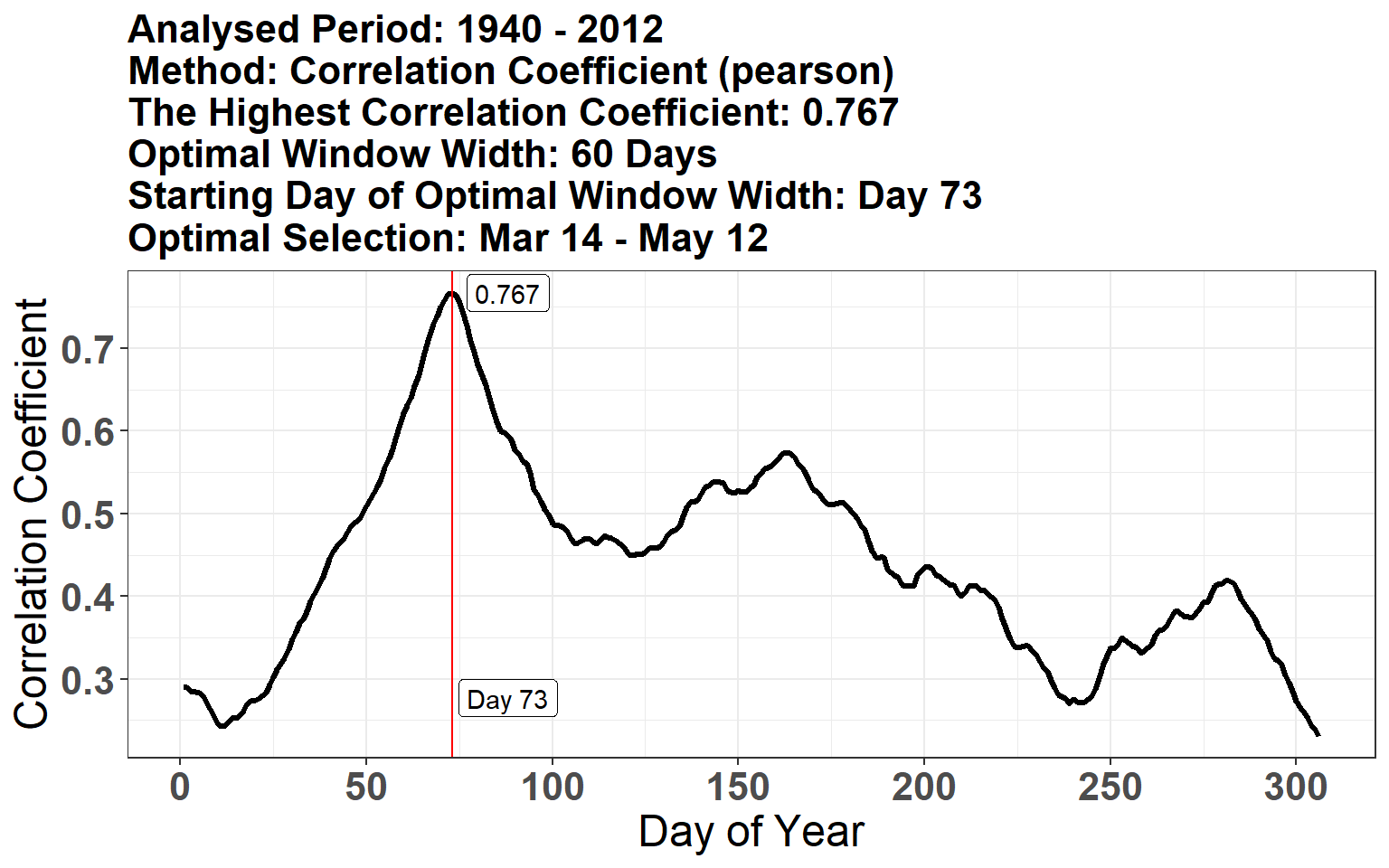# 1. Introduction

The dendroTools R package provides some novel dendroclimatological methods to analyse the relationship between tree-ring and environmental data. In this document we give details about how to use our package. All the data included in examples bellow is a part of the dendroTools R package.

Please note, the examples presented here are made computationally less intensive to satisfy the CRAN policy. You are invited to explore the full potential of our package by using the wide range of possible window widths.

# 2. The use of daily_response()

The daily_response() works by sliding a moving window through daily environmental data and calculates statistical metrics with one or more tree ring proxies. Possible metrics are correlation coefficient, coefficient of determination (r squared) or adjusted coefficient of determination (adjusted r squared). In addition to linear regression, it is possible to use nonlinear artificial neural network with Bayesian regularization training algorithm (brnn). In general, user can use a fixed or progressive window for calculations of moving averages. To use a fixed window, select its width by assigning an integer to the argument fixed_width. To use many different windows, define the lower_limit and upper_limit arguments. In this case, all window widths between the lower and upper limits will be considered. In the later text, window width representative of a specific day of year (DOY) is defined as the values for this particular day and number of subsequent days corresponding to window width. All calculated metrics are stored in a matrix, which is later used to find the optimal window (i.e. optimal consecutive sequence of days), that returns the highest calculated metric. This selection is later used to calculate temporal stability and cross validation.

## 2.1 Glimpse daily data

The glimpse_daily_data() is used for visual depiction of daily environmental data. Especially, it is useful to spot missing values and to evaluate the suitability of using specific daily data.

``````# Load the dendroTools R package
library(dendroTools)

data(LJ_daily_temperatures)
glimpse_daily_data(env_data = LJ_daily_temperatures, tidy_env_data = FALSE, na.color = "white")``````Figure 1: Glimpse of daily temperautres for meteorological station Ljubljana.

## 2.2 Example of analysing the relationship between mean vessel area (MVA) parameter and daily temperature data with fixed window width

In this example, we analyse the relationship between MVA and daily temperature data for wind width of 60 days (argument fixed_width = 60). Importantly, the row_names_subset argument is set to TRUE. This argument automatically subsets both data frames (i.e., environmental and tree-ring data) and keeps only matching years, which are used for calculations. To use this feature, years must be included as row names. All insignificant correlations are removed by setting the argument remove_insignificant to TRUE. The threshold for significance is set with the alpha argument.

``````# Load the dendroTools R package
library(dendroTools)

data(data_MVA)
data(LJ_daily_temperatures)

# Example with fixed width
example_fixed_width <- daily_response(response = data_MVA, env_data = LJ_daily_temperatures,
method = "cor", fixed_width = 60,
row_names_subset = TRUE, remove_insignificant = TRUE,
alpha = 0.05)``````
``example_fixed_width\$plot_extreme``Figure 2: The MVA parameter contains the optimal temperature signal from March 14 (DOY 73) to May 12 (DOY 132).

## 2.3 Example of analysing the statistical relationship between mean vessel area (MVA) parameter and daily temperature data for past and present

In the exploration of tree-climate relationships, researchers might be interested in how this relationship is different between past and present. The key argument for such analysis is subset_years, which defines the subset of years to be analysed. In the following example, we will analyse the relationship between MVA and daily temperature data for two periods, 1940 – 1980 and 1981 – 2010. All possible window widths between 50 and 70 days, including the previous year, will be considered. The latter is achieved by setting the previous_year argument to TRUE. Importantly, the row_names_subset argument is set to TRUE. This argument automatically subsets both data frames (i.e., environmental and tree-ring data) and keeps only matching years, which are used for calculations. To use this feature, years must be included as row names. All insignificant correlations are removed by setting the argument remove_insignificant to TRUE. The threshold for significance is set with the alpha argument.

After the function finish with all calculations, we plot the temporal patterns for past and present. In addition, we are interested in specific window width with span of 60 days (use plot_specific_window argument).

``````# Load the dendroTools R package
library(dendroTools)

data(data_MVA)
data(LJ_daily_temperatures)

# Example for past and present
example_MVA_past <- daily_response(response = data_MVA, env_data = LJ_daily_temperatures,
method = "cor", lower_limit = 50, upper_limit = 70,
row_names_subset = TRUE, previous_year = TRUE,
remove_insignificant = TRUE, alpha = 0.05,
plot_specific_window = 60, subset_years = c(1941, 1980))

example_MVA_present <- daily_response(response = data_MVA, env_data = LJ_daily_temperatures,
method = "cor", lower_limit = 50, upper_limit = 70,
row_names_subset = TRUE, previous_year = TRUE,
remove_insignificant = TRUE, alpha = 0.05,
plot_specific_window = 60, subset_years = c(1981, 2010))``````
``example_MVA_past\$plot_heatmap``